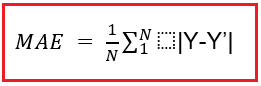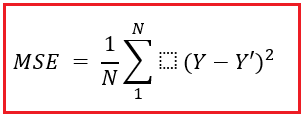# Model Evaluation for Regression in Machine Learning

## Model Evaluation for Regression in Machine Learning

In this article, I am going to discuss Model Evaluation for Regression in Machine Learning with Examples. Please read our previous article where we discussed Model Building and Validation in Machine Learning with Examples.

##### Model Evaluation for Regression in Machine Learning

We have multiple evaluation metrics for checking the model performance of a regression model –

##### Mean Absolute Error –

The absolute difference between actual and anticipated values is calculated using the MAE measure, which is a relatively basic statistic. Now you must locate your model’s MAE, which is essentially a mistake created by the model and is referred to as an error. Get the difference between the actual and anticipated values, which is an absolute error; however, we must first find the mean absolute of the entire dataset.Where,

1. N = number of data points
2. Y = actual output
3. Y’ = predicted output

The aim of a regression model should be to get the lowest MAE possible.

1. The MAE value you receive is in the same unit as the output variable.
2. It’s the most resistant to outliers.

Disadvantages – Because the graph of MAE is not differentiable, we must use differentiable optimizers such as gradient descent.

##### Mean Squared Error –

MSE is a widely used and straightforward statistic that accounts for a small change in mean absolute error. Finding the squared difference between the actual and anticipated value is defined as a mean squared error.

##### What does the MSE actually stand for?

It denotes the difference in squared values between actual and predicted values. The benefit of MSE is that we conduct squared to avoid the cancellation of negative terms.Where,

• N = number of data points
• Y = actual output
• Y’ = predicted output

Advantages – Because MSE’s graph is differentiable, it can readily be used as a loss function.

1. A squared unit of output is the result of computing MSE. For example, if the output variable is in meter(m), the output we get after computing MSE is in meter squared.
2. If the dataset contains outliers, the outliers are penalized the most, and the estimated MSE is larger. In other words, it is not robust against outliers, which was a benefit in MAE.
##### Root Mean Squared Error –

The acronym RMSE indicates that it is a simple square root of mean squared error.Advantages – Because the output value is in the same unit as the desired output variable, loss interpretation is simple.

Disadvantages – When compared to MAE, it is less resistant to outliers.

##### R-Squared (R2) Error –

The R2 score is a metric that measures the performance of your model, not the loss in terms of how many wells it performed. As we’ve seen, MAE and MSE are context-dependent, whereas the R2 score is context-independent.

So, using R squared, we can compare a model to a baseline model that none of the other metrics can provide. In classification problems, we have something similar called a threshold, which is set at 0.5. R2 squared calculates how much better a regression line is than a mean line.Where,

• SSr = Squared sum error of regression line
• SSm = Squared sum error of mean line

The R2 value increases as our regression line approach perfection. Furthermore, the model’s performance improves.

In the next article, I am going to discuss Classification and its Use Cases in Machine Learning with Examples. Here, in this article, I try to explain Model Evaluation for Regression in Machine Learning with Examples. I hope you enjoy this Model Evaluation for Regression in Machine Learning with Examples article.